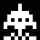Home Communities
IT Knowledge
Inspiration
Languages
EN

# Java - iterate through string

0 points
Created by:Burhan-Boyce
418

In this article, we would like to show you how to iterate through string in Java.

Quick solution:

``````String text = "ABC";

for (int i = 0; i < text.length(); i++) {
System.out.print(text.charAt(i));
}``````

## 1. Using for loop with String `charAt()`

In this example, we iterate through the `text` string and print each character.

``````public class Example {

public static void main(String[] args) throws Exception {
String text = "ABC";

for (int i = 0; i < text.length(); i++) {
System.out.print(text.charAt(i));
}
}
}``````

Output:

``ABC``

## 2. Using String `toCharArray()`

In this example,  we use String `toCharArray()` method to convert `text` String to the `characters` array. Then we use enhanced for-loop to iterate through the array.

``````public class Example {

public static void main(String[] args) throws Exception {
String text = "ABC";

char[] characters = text.toCharArray();

for (char ch : characters) {
System.out.print(ch);
}
}
}``````

Output:

``ABC``

## 3. Using String `split()`

In this example,  we use String `split()` method to convert `text` String to the `letters` array. Then we use enhanced for-loop to iterate through the array.

``````public class Example {

public static void main(String[] args) throws Exception {
String text = "ABC";

String[] letters = text.split("");

for (String letter : letters) {
System.out.print(letter);
}
}
}``````

Output:

``ABC``

## 4. Using Iterator

In this example, we use `StringCharacterIterator` class to iterate through the `text` string.

``````import java.text.CharacterIterator;
import java.text.StringCharacterIterator;

public class Example {

public static void main(String[] args) throws Exception {
String text = "ABC";
CharacterIterator iterator = new StringCharacterIterator(text);

while (iterator.current() != CharacterIterator.DONE)
{
System.out.print(iterator.current());
iterator.next();
}
}
}``````

Output:

``ABC``
Join to our subscribers to be up to date with content, news and offers.